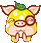登录 注册# 百花医的另一面 暴力奶妈暴击装出装表

## 大唐无双零百花医的另一面 暴力奶妈暴击装出装表《大唐无双零》玩家分享暴力百花医，暴击出装表，你还差多少件？

下面是暴击

敏捷=49% (1000点敏捷20+后面2900敏捷29)

马具=1%

金装=5% (至尊1+究穿1+究心3)

辅助=3%

药水=4% (爆击药水2+爆击合剂2%)

首饰=7% (真生杀套2+玉佩2+项链1+戒指1+指环1)

卓越=6% (防具5+武器1)

12属性=7% (防具5+武器2)

心法= 3% (神照1+菩提2)

光环=10%

双官阶=5%

总合为100%暴击

小编推荐：

下面是暴伤

马具5%

金装= 35% (究穿20+活力15)

卓越=30% (防具25+武器5)

首饰=30% (项链10+指环10+真生杀套10)

辅助=15%

12属性=30%

药水=5%

暴伤总合为150%

小编推荐：